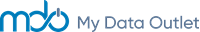Introduction

Key Performance Indicators (KPI) are industry-specific metrics reported by companies in the Airlines, Oil & Gas, Pharmaceutical, and Retail industries. Reporting of KPIs is not mandatory, yet many firms will report these metrics alongside their standard quarterly financial statements.

KPI metrics are available in the QA Direct I/B/E/S v2 database from Refinitiv, and we have conveniently made these items available on the MDO Platform, which can be easily accessed like any other fundamental data item using the FundamentalData() function to retrieve actual data or the FundamentalEstData() function to retrieve estimate data.

For a list of all available KPI metrics, please see the Appendix.

How to Retrieve KPI Data

On the MDO platform, you can view KPI items using the Data Item Lookup Table. For example, to get the mean estimate Total Number of Stores (NOS) for a firm, you can search for the data item as follows: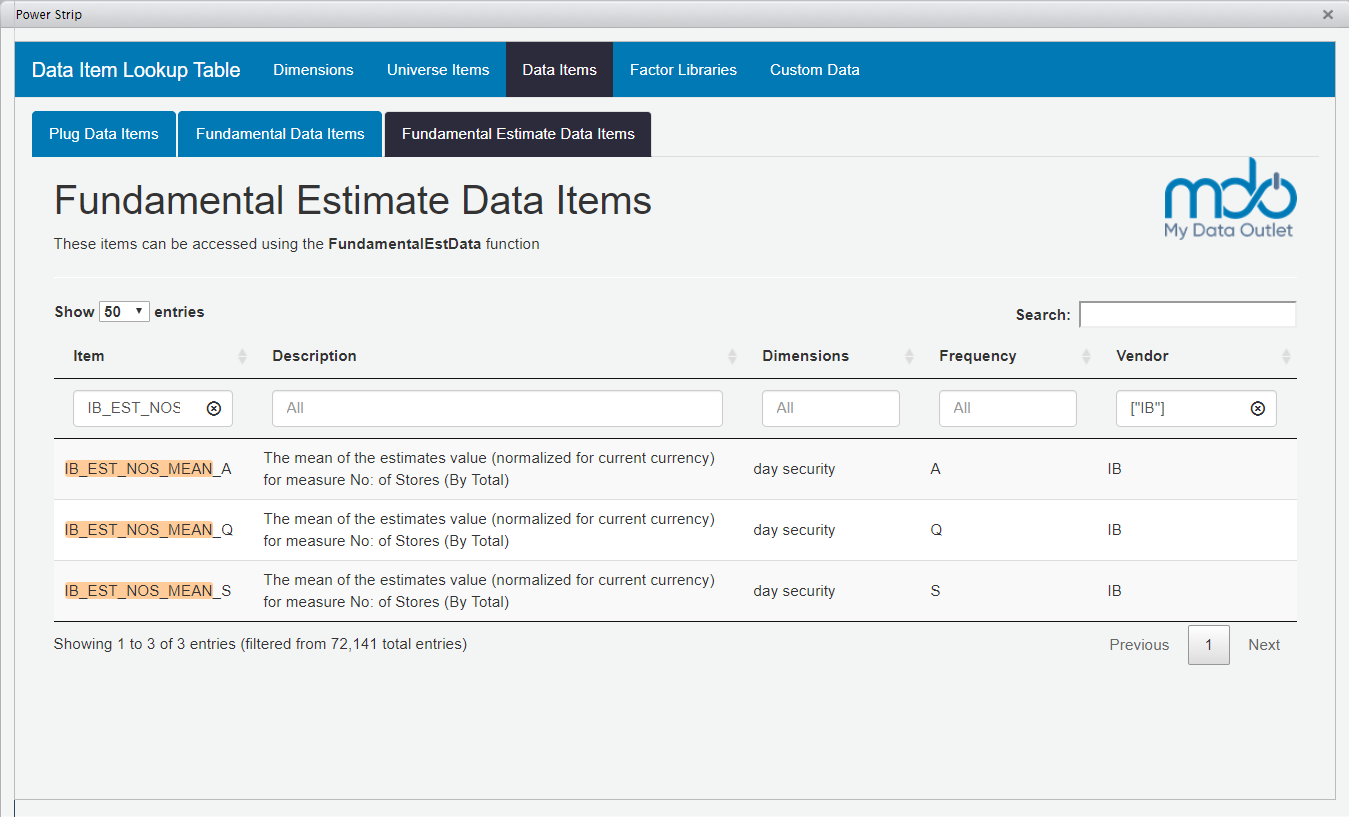Actual Data

Actual KPI data (those items that are reported by firms) have the following naming convention: IB_ACT_xyz_VALUE_frequency where:

• IB references the I/B/E/S v2 data from Refinitiv
• ACT and VALUE indicates that it’s actual value
• xyz is the KPI item mnemonic (e.g., ‘PRK’ for Passenger Revenue per Available Seat Mile, ‘NOS’ for Total Number of Stores, ‘OPD’ for Oil Production per Day, etc.)
• frequency is either ‘Q’ for quarterly, ‘S’ for semi-annual, or ‘A’ for annual.

Let’s retrieve and plot the actual Total Number of Stores (NOS) data for the following three retail firms: Target Corp (TGT-US), Costco Wholesale Corp (COST-US), and Gap Inc (GPS-US).

We will use the FundamentalData() function to retrieve this data, and we will set the period argument to zero to indicate that we want get data as of the most recent fiscal quarter.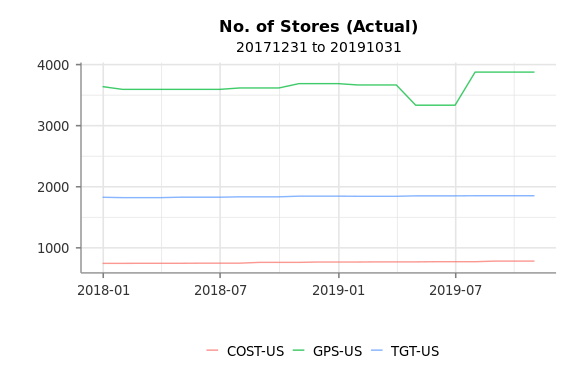[Source: I/B/E/S, Datastream]

Estimate Data

Similarly, the naming pattern for mean estimate data is: IB_EST_xyz_MEAN_frequency

You can retrieve either a broker-specific KPI estimate or a mean KPI estimate using the FundamentalEstData() function. For this example, we will retrieve the mean estimate NOS for those same three firms. Here, the period = 1 argument indicates that you want to look forward one quarter (i.e., retrieve estimate data for FQ1).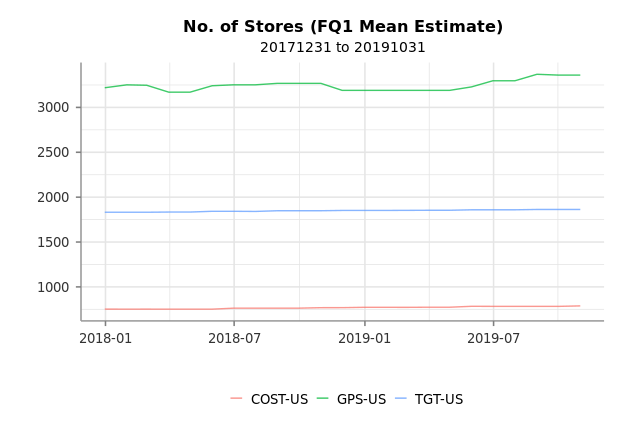[Source: I/B/E/S, Datastream]

KPI Quant Factor Examples

On the MDO platform, you can easily build factors using any data item, and we’ve already created a few factors that contain KPI data to help you get started:

• For the Airlines industry, we’ve created a factor named MDQ_KPI_AIRLINES_GROSS_PROFIT_ASM_YLD, which is an estimated gross profit yield measure that is calculated as the average estimated revenue per available seat mile (ASM) less cost per ASM over the next four quarters multiplied by the total ASM over the prior four quarters divided by the current market capitalization.
• For the Oil & Gas industry, we have a factor named MDQ_KPI_OILGAS_EXPL_EXP_SURP, which simply measures the surprise in the exploration expense between analyst expectations and actual reported expense value.
• For the Retail industry, we have MDQ_KPI_RETAIL_SALES_GROWTH_SQFT, which measures the percent change in net sales per average square foot from four quarters ago.

Factor Analysis

For this demonstration, we will investigate the MDQ_KPI_RETAIL_SALES_GROWTH_SQFT factor. Let’s start by retrieving the constituents of the Russell 1000 index.

We will need to filter out firms that don’t report retail sales data.

Next, we will run the QFPerformance() function on our factor, which will calculate information coefficients of the factor over various time horizons and the historical performance of the factor quantiles. The n = 4 argument indicates that you want to quartile the factor and wt = "cap" indicates that you want to use market capitalization-weighted returns (as opposed to equal-weighted returns).

The QFPerformance() function returns a performance object that contains a results slot of various performance measures. The summary report below is one of the more important tables and shows the annualized risk-adjusted returns of each quantile over the entire time period.

factor quantile Total Return Std Dev Sharpe Ratio
MDQ_KPI_RETAIL_SALES_GROWTH_SQFT 1 0.1546353 0.1719891 0.8990996
MDQ_KPI_RETAIL_SALES_GROWTH_SQFT 2 0.2223635 0.1699145 1.3086785
MDQ_KPI_RETAIL_SALES_GROWTH_SQFT 3 0.1165608 0.1956126 0.5958758
MDQ_KPI_RETAIL_SALES_GROWTH_SQFT 4 0.0793771 0.2486463 0.3192370

[Source: I/B/E/S, Datastream]

The following plot shows the average rank correlations of the factor values and their subsequent 1-, 2-, 3-, …, 12-month returns.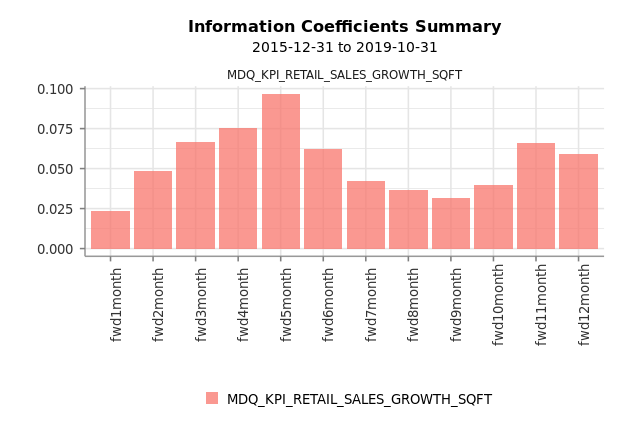[Source: I/B/E/S, Datastream]

The next report shows the cumulative returns of each quantile over time.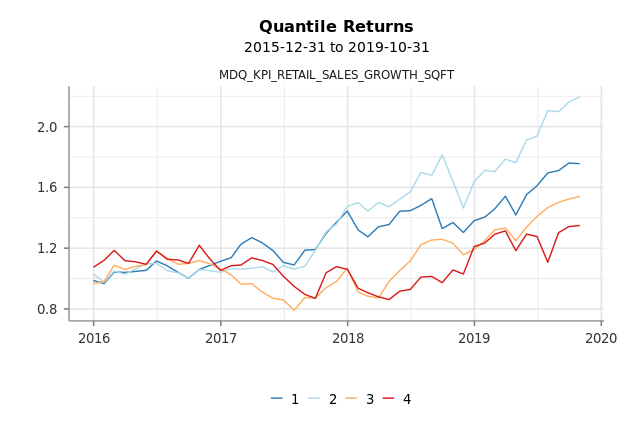[Source: I/B/E/S, Datastream]

Finally, the long-short performance of the top quantile (1st quartile) versus the bottom quantile (4th quartile) is shown below.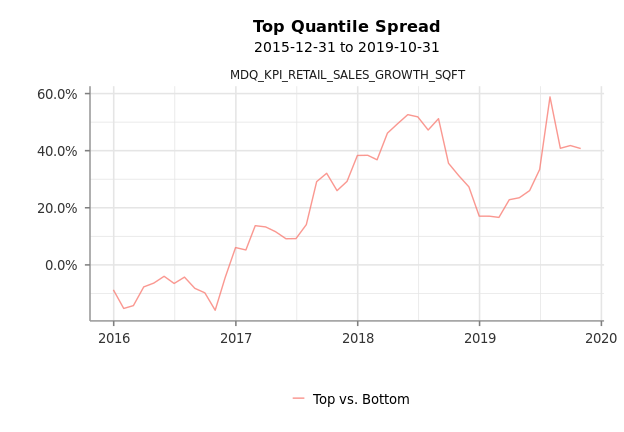[Source: I/B/E/S, Datastream]

Appendix

Airlines

• ASM = Available Seat Miles; total number of revenue-available seats times the numbers of miles traveled
• RPM = Revenue Passenger Miles; total number of revenue-generating seats times the numbers of miles traveled
• PLF = Passenger Load Factor; it is the RPM as a percent of ASM
• CPA = Operating Expense per Available Seat Mile
• PRK = Passenger Revenue per Available Seat Mile

Oil & Gas

• DCF = Distributable Cash Flow
• OPD = Oil Production per Day
• TPD = Total Production per Day
• GPD = Gas Production per Day
• RPO = Realized Oil Price
• RPG = Realized Gas Price
• EBX = EBITDAX; Earnings Before Interest Taxes Depreciation Amortization and Exploration Expense
• NPP = Natural Gas Liquids Production per Day
• MCX = Maintenance Capital Expenditure
• LOE = Lease Operating Expense
• EXP = Exploration Expense
• TPP = Total Production per Day
• PTX = Production Tax
• RZP = Realized Price (BOE)
• PEX = Production Expense

Retail

• NOS = Total Number of Stores
• FLS = Total Floor Space (in square feet)
• NOO = Number of Stores Opened
• RES = Retails Sales
• NAS = Net Sales per Average Square Foot
• NSC = Number of Stores Closed or Relocated